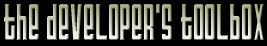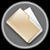This section of the archives stores flipcode's complete Developer Toolbox collection, featuring a variety of mini-articles and source code contributions from our readers.Input Engine   Submitted byThis is a small input engine I made. It translates input data by using key bindings, and execute the relevant code. The program registers controllers to the input engine. A controller is a function that a key can be bound to. When a controller is registered it is associated with a controller ID, which is what the keys are also associated with. When a key is pressed, the engine looks up the associated controller, and runs it.

This code is free to use anyway you like, but it would be fun if you let me know if you do. Fredrik Redgňrd
mrfrippe@writeme.com
www.fredriksoftware.com

 ```/******************************************************************************/ /* Input Engine Concept Code */ /* - Fredrik Redgňrd */ /******************************************************************************/#include /// /// This is a shortened version of my input engine /// the keymappings can be loaded from a configuration /// file, and updated from an options screen /// /// The keybindings define what each key does, by /// assigning that key a controller ID. /// When the key is pressed, the controller ID is /// found in the list of controllers, and /// the controller code is executed /// /// I don't know how fast this is, or if there are any /// optimizations that can be done. /// /// To use it, you need to make controller IDs for each /// function that can be bound to keys /// /// by keys I also mean mouse buttons, function keys, /// timers, script interupts. /// /// how you collect this input is up to you. /// #define ERROR_CONTROLLER_NOT_FOUND ((InputController*)(-1))#define CID_NONE 0 #define CID_MOVEUP 1 #define CID_MOVEDOWN 2 #define CID_MOVELEFT 3 #define CID_MOVERIGHT 4/// Base Class for the input controllers class InputController { public: virtual void Down() = 0; /// key down virtual void Up() = 0; /// key up };/// Move controller, just a simple test class MoveController : public InputController { public: void Down() { /// key bound to this function has been pressed, /// do something cout << m_value; } void Up() { /// in this test, nothing happens when the key is released }; MoveController( char *value ) { m_value = value; } private: char *m_value; };/// Node type for the controller list /// each controller has an ID defined in the beginning /// of this file. typedef struct ControllerNode { ControllerNode *next; int controller_id; InputController *controller; } ControllerNode;/// pointer to the first node in the list ControllerNode *first = 0;/// Registers a controller for use by adding /// it to the list void MakeController( int controller_id, InputController *controller ) { ControllerNode *cn = new ControllerNode; cn->next = first; cn->controller = controller; cn->controller_id = controller_id; first = cn; }/// the key bindings ( this test only uses 10 keys ) /// keybindings should bind all keys, function keys, mouse buttons, etc /// to controller IDs, this is used to translate a keypress /// to a controller function int keybindings = { CID_MOVEUP, //key 0 = controller UP CID_MOVEDOWN, //key 1 = controller DOWN CID_MOVELEFT, //key 2 = controller LEFT CID_MOVERIGHT, //key 3 = controller RIGHT CID_MOVEUP, //key 4 = controller UP CID_MOVELEFT, // ... CID_MOVEUP, CID_NONE, //key 7 is not bound to anything CID_NONE, // CID_MOVEDOWN };/// Finds the controller bound to the key /// that was pressed InputController *GetController( int key ) { if (keybindings[key] == CID_NONE) return NULL; ControllerNode *cn = first; while (cn != 0) { if (keybindings[key] == cn->controller_id) return cn->controller; cn = cn->next; } return ERROR_CONTROLLER_NOT_FOUND; }///Theese functions are used where ever input is collected void KeyDown( int key ) { InputController *ic; ic = GetController(key); if ((ic!=NULL) && (ic!=ERROR_CONTROLLER_NOT_FOUND)) ic->Down(); } void KeyUp( int key ) { InputController *ic; ic = GetController(key); if ((ic!=NULL) && (ic!=ERROR_CONTROLLER_NOT_FOUND)) ic->Up(); }/// test implementation int main(int argc, char* argv[]) { /// The MakeController calls should be made in the initiation code of the /// different systems, like camera movement, player position etc. /// I also use the MoveController more than once, but you can use /// different controllers for each function /// the objects are destroyed by the input engine when they are not needed /// anymore MakeController( CID_MOVEUP, new MoveController("move up") ); MakeController( CID_MOVEDOWN, new MoveController("move down") ); MakeController( CID_MOVELEFT, new MoveController("move left") ); MakeController( CID_MOVERIGHT, new MoveController("move right") ); /// temporary pointer for walking in the list InputController *ic; for (int i=0; i<10; i++) { cout << "key " << i << " = "; ic = GetController(i); /// see if a controller is bound to this key if ((ic != NULL) && (ic != ERROR_CONTROLLER_NOT_FOUND)) { ic->Down(); /// if so, press the key ic->Up(); /// and release it } else if (ic == ERROR_CONTROLLER_NOT_FOUND) { cout << "error, controller not found"; } cout << endl; } return 0; }/*/ Output: key 0 = move up key 1 = move down key 2 = move left key 3 = move right key 4 = move up key 5 = move left key 6 = move up key 7 = key 8 = key 9 = move down Press any key to continue/*/ ```